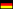TUHH / Institute of Mathematics / Staff / Katharina Klioba, M. Sc.# Katharina Klioba, M. Sc.Am Schwarzenberg-Campus 3
Building E
D-21073 Hamburg
Room: 3.078
Phone: +49 40 42878 3058

### Office Hours

summer term 23: on wednesdays 1.45-2.45pm in E3.078,
by appointment only during lecture-free time and for online office hours

### Doctoral Thesis

Approximation of Operator Semigroups (Joint research with Dr. Christian Seifert)

$C_0$-semigroups provide a functional analytic framework allowing to solve a large class of time-dependent partial differential equations (PDE) numerically using methods for ordinary differential equations (ODE). The PDE is rewritten as the abstract Cauchy problem (ACP) $$\dot{u}(t) =Au(t),~~t\ge 0\\ u(0)=u_0 \in X,$$ where $A$ generates a strongly continuous semigroup $(T(t))_{t\ge 0}$ on a Banach space $X$. Although the solution of the ACP is known to be $u(t)=T(t)u_0$, it is in general not feasible to determine the semigroup $T(t)$ directly. This is where numerical methods for ODEs come into play: Space discretisation schemes (such as finite differences or, if $\dim X >1$, finite elements) can be applied to $A$ resulting in approximations $A_m$ generating approximating semigroups $(T_m(t))_{t \ge 0}$ on often finite-dimensional Banach spaces $X_m$. The Trotter-Kato theorem [1,2] answers the question under which conditions this implies convergence of the semigroups $(T_m(t))_{t\ge 0}$. Quantified versions of it provide convergence rates on dense subspaces . One aim of my research is to provide a quantified Trotter-Kato-like theorem for the case of form-induced generators, i.e. when $X$ is a Hilbert space and the generator is only given weakly by some form $a:V \times V \to \mathbb{C}$ acting on a densely embedded Hilbert space $V \hookrightarrow X$. That is, $$\langle Au,v \rangle = a(u,v)~~~~~\forall u \in D(A) \cap V, v \in V.$$ Conditions are investigated on the approximating forms $a_m :=a|_{V_m}$ and the finite-dimensional approximating spaces $V_m \subseteq V$ such that semigroup convergence of a certain rate is attained. Furthermore, this approximation framework shall be generalized to the case where $V$ is not a subspace of $X$ but they are only related via a linear and bounded mapping $j:V \to X$ with dense range. This allows to treat the Dirichlet-to-Neumann operator, which, for instance, arises in Electrical Impedance Tomography. Another generalization of interest to my research are stochastic evolution equations, where the generator $A$ is no longer deterministic but depends on random input. Hence, approximation is not only performed in space and time but also in randomness, employing e.g. polynomial chaos approximation (PCE) techniques. This requires generalizing the approximation framework as well as deriving convergence rates for multi-dimensional PCE approximations .

References
1. H. F. Trotter, Approximation of semi-groups of operators, Pacific J. Math. 8 (1958), 887-919.
2. T. Kato, Perturbation Theory for Linear Operators, Springer-Verlag, New York, 1976.
3. K. Ito and F. Kappel. The Trotter-Kato Theorem and Approximation of PDEs, Mathematics of Computation 67, no. 221 (1998), 21-44.
4. E. Frick, D. Dahl, K. Klioba, C. Seifert, M. Lindner, C. Schuster, Quantitative Error Bounds of Polynomial Chaos Expansion and their Application to Resonant Systems, 2019, Institute of Mathematics, TUHH